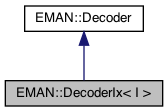EMAN2
EMAN::DecoderIx< I > Struct Template Reference

`#include <eerio.h>`

Inheritance diagram for EMAN::DecoderIx< I >:[legend]
Collaboration diagram for EMAN::DecoderIx< I >:[legend]

## Public Member Functions

unsigned int num_pix () const override

unsigned int x (unsigned int count, unsigned int sub_pix) const override

unsigned int y (unsigned int count, unsigned int sub_pix) const override

unsigned int x (unsigned int count, unsigned int sub_pix) const

unsigned int y (unsigned int count, unsigned int sub_pix) constPublic Member Functions inherited from EMAN::Decoder
auto operator() (unsigned int count, unsigned int sub_pix) constPublic Attributes inherited from EMAN::Decoder
const unsigned int camera_size_bits = 12

const unsigned int camera_size = 1 << camera_size_bits

## Detailed Description

template<unsigned int I>
struct EMAN::DecoderIx< I >

Definition at line 135 of file eerio.h.

## ◆ num_pix()

template<unsigned int I>
 unsigned int EMAN::DecoderIx< I >::num_pix
overridevirtual

Implements EMAN::Decoder.

Definition at line 142 of file eerio.h.

142 {
143// 4096 * 1 // 4k
144// 4096 * 2 // 8k
145// 4096 * 4 // 16k
146 return camera_size * (1 << I);
147 }
const unsigned int camera_size
Definition: eerio.h:126

## ◆ x() [1/2]

 unsigned int EMAN::DecoderIx< 0 >::x ( unsigned int count, unsigned int sub_pix ) const
inlinevirtual

Implements EMAN::Decoder.

Definition at line 164 of file eerio.h.

164 {
165// count & ((1 << 12) - 1)
166 return count & (camera_size - 1);
167 }

## ◆ x() [2/2]

template<unsigned int I>
 unsigned int EMAN::DecoderIx< I >::x ( unsigned int count, unsigned int sub_pix ) const
overridevirtual

Implements EMAN::Decoder.

Definition at line 150 of file eerio.h.

150 {
151// (((count & 4095) << 2) | ((sub_pix & 3) ^ 2)) // 16k
152// (((count & 4095) << 1) | ((sub_pix & 3) ^ 2) >> 1) // 8k
153 return (DecoderIx<0>().x(count, sub_pix) << I) | (((sub_pix & 3) ^ 2) >> (2 - I));
154 }
unsigned int x(unsigned int count, unsigned int sub_pix) const override
Definition: eerio.h:150

References x.

## ◆ y() [1/2]

 unsigned int EMAN::DecoderIx< 0 >::y ( unsigned int count, unsigned int sub_pix ) const
inlinevirtual

Implements EMAN::Decoder.

Definition at line 170 of file eerio.h.

170 {
171// count >> 12
172 return count >> camera_size_bits;
173 }
const unsigned int camera_size_bits
Definition: eerio.h:125

## ◆ y() [2/2]

template<unsigned int I>
 unsigned int EMAN::DecoderIx< I >::y ( unsigned int count, unsigned int sub_pix ) const
overridevirtual

Implements EMAN::Decoder.

Definition at line 157 of file eerio.h.

157 {
158// (((count >> 12) << 2) | ((sub_pix >> 2) ^ 2)) // 16k
159// (((count >> 12) << 1) | ((sub_pix >> 2) ^ 2) >> 1) // 8k
160 return (DecoderIx<0>().y(count, sub_pix) << I) | (((sub_pix >> 2) ^ 2) >> (2 - I));
161 }
unsigned int y(unsigned int count, unsigned int sub_pix) const override
Definition: eerio.h:157

References y.

The documentation for this struct was generated from the following file: